Pythagorean theorem. From your study of Mathematics, Volume 1, you should recall that this theorem is stated as follows: In a right triangle, the square of the length of the hypotenuse (longest side) is equal to the sum of the squares of the lengths of the other two sides."> Distance Between Two PointsCustom SearchDISTANCE BETWEEN TWO POINTS The distance between two points, P, and P2, can be expresssed in terms of their coordinates by using the Pythagorean theorem. From your study of Mathematics, Volume 1, you should recall that this theorem is stated as follows: In a right triangle, the square of the length of the hypotenuse (longest side) is equal to the sum of the squares of the lengths of the other two sides.   Let the coordinates of P, be (x),y,) and let those of P2 be (X2,Y2), as shown in figure 1-2. By the Pythagorean theorem,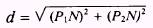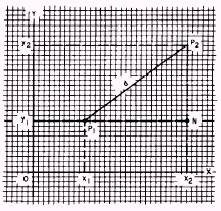Figure 1-2.-Distance between two points. where P1N represents the distance between x, and x2, P2N represents the distance between y1 and y2, and d represents the distance from P1 to P2. We can express the length of P1N in terms of x, and x2 and the length of P2Nin terms of y1 and y2 as follows: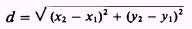Although we have demonstrated the formula for the first quadrant only, it can be proven for all quadrants and all pairs of points. EXAMPLE: In figure 1-2, x, = 2,x2 = 6, y, = 2, and y2 = 5. Find the length of d. SOLUTION: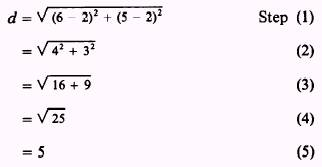This result could have been foreseen by observing that triangle P1NP2 is a 3-4-5 triangle. EXAMPLE: Find the distance between P1 (4,6) and P2 (10,4). SOLUTION: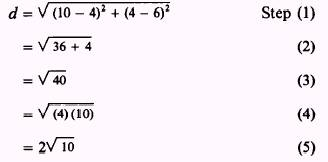DIVISION OF A LINE SEGMENT Many times you may need to find the coordinates of a point that is some known fraction of the distance between P1 and P2. In figure 1-3, P is a point lying on the line joining P1 and P2 so that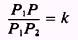If P should lie 1/4 of the way between P1 and P2, then k would equal 1/4. Triangles P1MP and P1NP2 are similar. Therefore,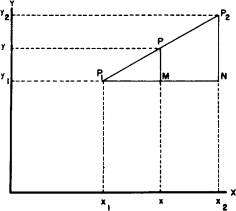Figure 1-3.-Division of a line segment.     Since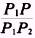is the ratio that defines k1 then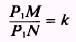Therefore, P1M = k(PIN) Refer again to figure 1-3 and observe that P1N is equal to X2- x,. Likewise, P1M is equal to x - x1. When you replace P1M and P1N with their equivalents in terms of x, the preceding equation becomes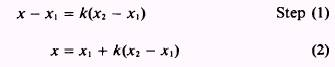By similar reasoning,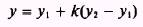The x and y found as a result of the foregoing discussion are the coordinates of the desired point, whose distances from P1 and P2 are determined by the value of k. EXAMPLE: Find the coordinates of a point 1/4 of the way from P1(2,3) to P2(4,1). SOLUTION: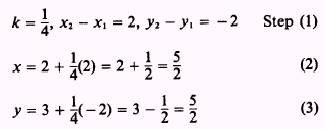Therefore, point P is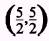. When the midpoint of a line segment is to be found, the value of (Io is 1/2. Therefore,such that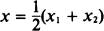By similar reasoning,EXAMPLE: Find the midpoint of the line between P1(2,4) and P2(4,6). SOLUTION: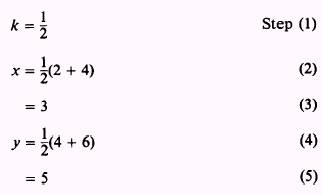Therefore, the midpoint is (3,5).Integrated Publishing, Inc. - A (SDVOSB) Service Disabled Veteran Owned Small Business